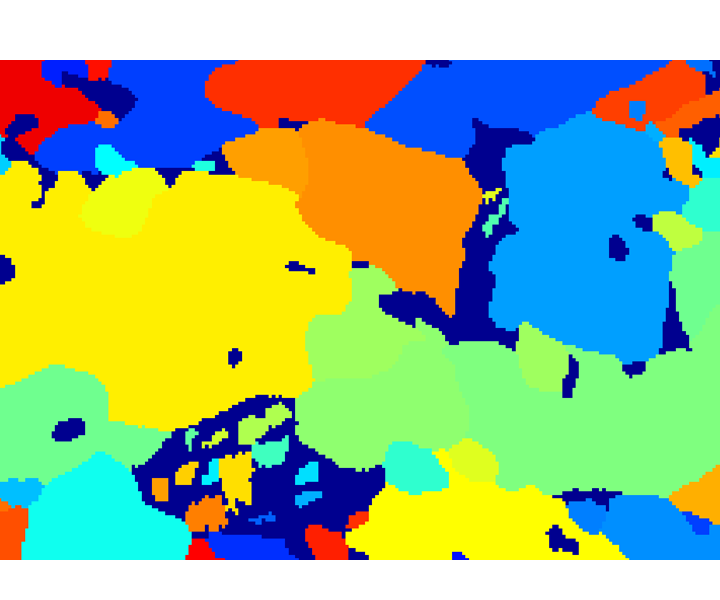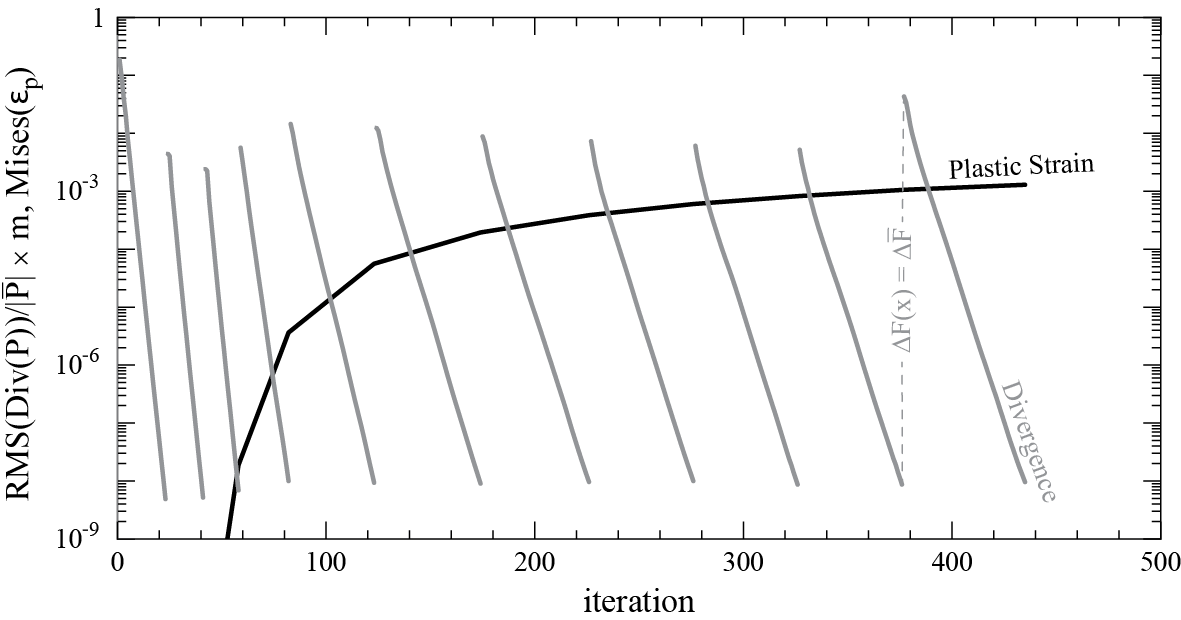# 1.3. Spectral Solver



## Introduction

The present implementation is based on the works by P. Suquet  and R.A. Lebensohn . The »basic scheme« included in DAMASK is described in  and . As an faster and more robust variant of the originally proposed fix-point algorithm, DAMASK includes also a variant using any suitable non-linear solver provided by PETSc. As an second alternative, an version using the Polarization field is included.## Solver variants

### Polarization## Convergence criterion

Since the iterative »basic scheme« (using either the fix-point algorithm or a non-linear solver provided by PETSc) strictly maintains compatibility, it is only necessary to achieve (static) equilibrium. As suitable convergence criterion Moulinec and Suquet  suggested

\begin{alignat}{1} \varepsilon_\text{eq} \ge \frac{\operatorname{RMS}(\operatorname{Div} \textbf{P}(\textbf x)) }{|| \bar{\textbf{P}}||} \times \text{m} \label{eq:convergenceCriterion} \end{alignat}

based on the the root mean square (RMS) value of $\operatorname{Div}(\tnsr P)$, which can be conveniently calculated in Fourier space employing Parseval's theorem.

### Corrections for physical size and grid resolution

The magnitude of the divergence depends on the distance between two neighboring Fourier points, i.e. for the same number of Fourier points but different physical dimension the iterative scheme will stop after different numbers of iterations. To correct for a dependence on the size and resolution of the volume element (VE), the spectral solver can be instructed to rescale all grid point distances by the same factor such that for the dimension with the medium pixel resolution a grid point distance of

1. as before (uncorrected)
2. 1 meter / grid point number (fixed dimension)
3. 1 meter (fixed grid point distance, default)
4. 1 meter / square root of grid point number (dimension and square root resolution corrected)

is obtained.

### Fixed grain structure at varying VE size and resolution

As example we take a cubic grain structure (included as example in DAMASK) at either 1 or 10 m side length and discretized by either 163, 323, or 643 Fourier points (FPs).(a) 163(b) 323(c) 643
Figure 1: Periodic crystal of 20 grains (included in DAMASK as example) discretized at different resolutions.

Figure 2 presents the normalized divergence (see eq. \eqref{eq:convergenceCriterion}) for all four above rescaling options.(a) uncorrected(b) fixed dimension(c) fixed grid point distance(d) dimension and square root of resolution corrected
Figure 2: Evolution of convergence criterion with iterations for the exemplary polycrystal at various resolutions (basic fix-point rev. 2232, first step of tensile test load case).

### Constant Grains per Fourier Point(a) original(b) 33 copies(c) 36 copies
Figure 3: Polycrystal of 20 grains at different resolution periodic copies(b) fixed dimension(c) fixed grid point distance(d) dimension and square root of resolution corrected
Figure 4: Divergence over iterations for periodic copies of VE (basic fix-point rev. 2232, first step of tensile test load case).

### Convergence behavior(a) Polycrystal, colors indicate orientation(b) Dual-phase steel, martensite in dark blue, ferrite grains indicated by other colors
Figure 5: Input Data(a) Polycrystal(b) Dual-phase steel
Figure 6: Evolution of divergence and plastic strain (basic PETSc rev. 2232)P. Eisenlohr, M. Diehl, R.A. Lebensohn, F. Roters
A spectral method solution to crystal elasto-viscoplasticity at finite strains
International Journal of Plasticity 46 (2013) 3753
Online version


M. Diehl
A spectral method using fast Fourier transform to solve elastoviscoplastic mechanical boundary value problems
Diploma Thesis, TU München (2010)


H. Moulinec, P. Suquet
A numerical method for computing the overall response of nonlinear composites with complex microstructure
Computer Methods in Applied Mechanics and Engineering 157 (1998) 6994
Online version


R.A. Lebensohn
N-site modeling of a 3D viscoplastic polycrystal using Fast Fourier Transform
Acta Materialia 49 (2001) 27232737
Online version


P. Shanthraj, P. Eisenlohr, M. Diehl, F. Roters
Numerically robust spectral methods for crystal plasticity simulations of heterogeneous materials
International Journal of Plasticity 66 (2015) 3145
Online version

This topic: Documentation > Background > SpectralSolver
Topic revision: 03 May 2018, JaeyongJung
Copyright by the contributing authors. All material on this collaboration platform is the property of the contributing authors.
Ideas, requests, problems regarding DAMASK? Send feedback
§ Imprint § Data Protection